# Multiple Linear Regression - Teradata Warehouse Miner

## Teradata Warehouse Miner User Guide - Volume 3Analytic Functions

Product
Release Number
5.4.5
Published
February 2018
Language
English (United States)
Last Update
2018-05-04
dita:mapPath
yuy1504291362546.ditamap
dita:ditavalPath
ft:empty
dita:id
B035-2302
Product Category
Software

Multiple Linear Regression analysis attempts to predict, or estimate, the value of a dependent variable as a linear combination of independent variables, usually with a constant term included. That is, it attempts to find the b-coefficients in the following equation in order to best predict the value of the dependent variable y based on the independent variables x 1 to x n .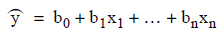## Multiple Linear Regression

The best values of the coefficients are defined to be the values that minimize the sum of squared error values: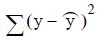over all the observations.

Note that this requires that the actual value of y be known for each observation, in order to contrast it with the predicted value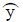. This technique is called “least-squared errors.” It turns out that the b-coefficient values to minimize the sum of squared errors can be solved using a little calculus and linear algebra. It is worth spending just a little more effort in describing this technique in order to explain how Teradata Warehouse Miner performs linear regression analysis. It also introduces the concept of a cross-products matrix and its relatives the covariance matrix and the correlation matrix that are so important in multivariate statistical analysis.

In order to minimize the sum of squared errors, the equation for the sum of squared errors is expanded using the equation for the estimated y value, and then the partial derivatives of this equation with respect to each b-coefficient are derived and set equal to 0. (This is done in order to find the minimum with respect to all of the coefficient values). This leads to n simultaneous equations in n unknowns, which are commonly referred to as the normal equations. For example: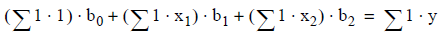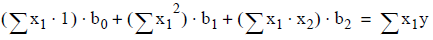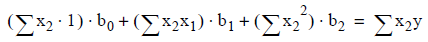The equations above have been presented in a way that gives a hint to how they can be solved using matrix algebra (i.e., by first computing the extended Sum-of-Squares-and-Cross-Products (SSCP) matrix for the constant 1 and the variables x 1 , x 2 and y). By doing this one gets all of the ∑ terms in the equation. Teradata Warehouse Miner offers the Build Matrix function to build the SSCP matrix directly in the Teradata database using generated SQL. The linear regression module then reads this matrix from metadata results tables and performs the necessary calculations to solve for the least-squares b-coefficients. Therefore, that part of constructing a linear regression algorithm that requires access to the detail data is simply the building of the extended SSCP matrix (i.e., include the constant 1 as the first variable), and the rest is calculated on the client machine.

There is, however, much more to linear regression analysis than building a model (i.e., calculating the least-squares values of the b-coefficients). Other aspects such as model diagnostics, data quality reports and stepwise regression are described in the following sections.